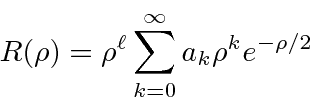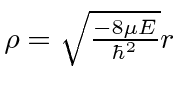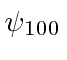## Hydrogen

The Hydrogen (Coulomb potential) radial equation is solved by finding the behavior at large, then finding the behavior at small, then using a power series solution to getwith. To keep the wavefunction normalizable the power series must terminate, giving us our energy eigenvalue condition.Hereis called the principle quantum number and it is given bywhereis the number of nodes in the radial wavefunction. It is an odd feature of Hydrogen that a radial excitation and an angular excitation have the same energy.

So a Hydrogen energy eigenstateis described by three integer quantum numbers with the requirements that,and also an integer, and. The ground state of Hydrogen isand has energy of -13.6 eV. We compute several of the lowest energy eigenstates.

The diagram below shows the lowest energy bound states of Hydrogen and their typical decays.Jim Branson 2013-04-22